## Example 57.10 Combining Correlation Coefficients

This example combines sample correlation coefficients computed from a set of imputed data sets by using Fisher’stransformation.

Fisher’stransformation of the sample correlationis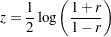The statisticis approximately normally distributed with mean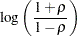and variance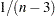, where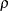is the population correlation coefficient andis the number of observations.

The following statements use the CORR procedure to compute the correlationand its associated Fisher’sstatistic between variables Oxygen and RunTime for each imputed data set. The ODS statement is used to save Fisher’sstatistic in an output data set.

```proc corr data=outmi fisher(biasadj=no);
var Oxygen RunTime;
by _Imputation_;
ods output FisherPearsonCorr= outz;
run;
```

The following statements display the number of observations and Fisher’sstatistic for each imputed data set in Output 57.10.1:

```proc print data=outz;
title 'Fisher''s Correlation Statistics';
var _Imputation_ NObs ZVal;
run;
```

Output 57.10.1 OutputStatistics
 Fisher's Correlation Statistics

Obs _Imputation_ NObs ZVal
1 1 31 -1.27869
2 2 31 -1.30715
3 3 31 -1.27922
4 4 31 -1.39243
5 5 31 -1.40146

The following statements generate the standard error associated with thestatistic,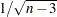:

```data outz;
set outz;
StdZ= 1. / sqrt(NObs-3);
run;
```

The following statements use the MIANALYZE procedure to generate a combined parameter estimateand its variance, as shown in Output 57.10.2. The ODS statement is used to save the parameter estimates in an output data set.

```proc mianalyze data=outz;
ods output ParameterEstimates=parms;
modeleffects ZVal;
stderr StdZ;
run;
```

Output 57.10.2 Combining Fisher’sStatistics
The MIANALYZE Procedure

Parameter Estimates
Parameter Estimate Std Error 95% Confidence Limits DF Minimum Maximum Theta0 t for H0:
Parameter=Theta0
Pr > |t|
ZVal -1.331787 0.200327 -1.72587 -0.93771 330.23 -1.401459 -1.278686 0 -6.65 <.0001

In addition to the estimate for, PROC MIANALYZE also generatesconfidence limits for,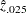and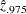. The following statements print the estimate andconfidence limits forin Output 57.10.3:

```proc print data=parms;
title 'Parameter Estimates with 95% Confidence Limits';
var Estimate LCLMean UCLMean;
run;
```

Output 57.10.3 Parameter Estimates withConfidence Limits
 Parameter Estimates with 95% Confidence Limits

Obs Estimate LCLMean UCLMean
1 -1.331787 -1.72587 -0.93771

An estimate of the correlation coefficient with its correspondingconfidence limits is then generated from the following inverse transformation as described in the section Correlation Coefficients: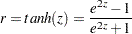for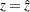,, and.

The following statements generate and display an estimate of the correlation coefficient and itsconfidence limits, as shown in Output 57.10.4:

```data corr_ci;
set parms;
r=       tanh( Estimate);
r_lower= tanh( LCLMean);
r_upper= tanh( UCLMean);
run;
proc print data=corr_ci;
title 'Estimated Correlation Coefficient'
' with 95% Confidence Limits';
var r r_lower r_upper;
run;
```

Output 57.10.4 Estimated Correlation Coefficient
 Estimated Correlation Coefficient with 95% Confidence Limits

Obs r r_lower r_upper
1 -0.86969 -0.93857 -0.73417